# “StackLLaMA”: 用 RLHF 训练 LLaMA 的手把手教程

ChatGPTGPT-4Claude 语言模型 之强大，因为它们采用了 基于人类反馈的强化学习 (Reinforcement Learning from Human Feedback, RLHF) 来使之更符合我们的使用场景。

• 有监督的微调 (Supervised Fine-tuning，SFT)。
• 奖励 / 偏好建模 (Reward / preference modeling，RM)。
• 基于人类反馈的强化学习 (RLHF)。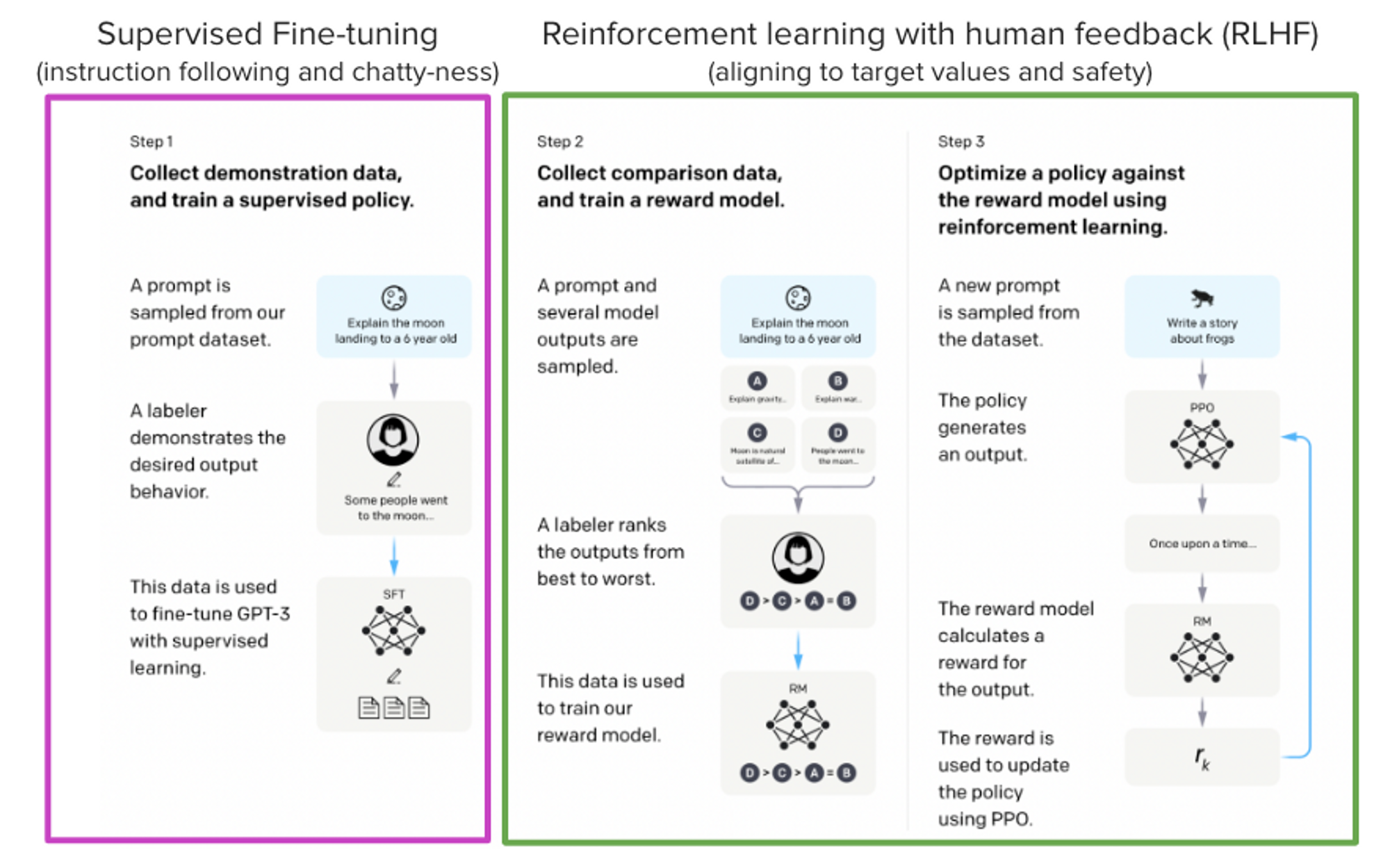## Stack Exchange 数据集

``````score = log2 (1 + upvotes) rounded to the nearest integer, plus 1 if the questioner accepted the answer (we assign a score of −1 if the number of upvotes is negative).
``````

## 高效训练策略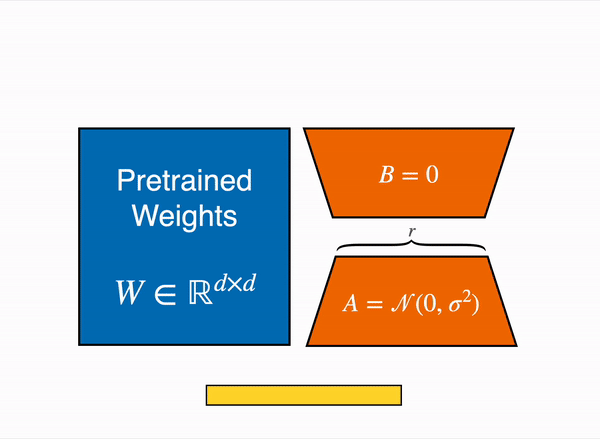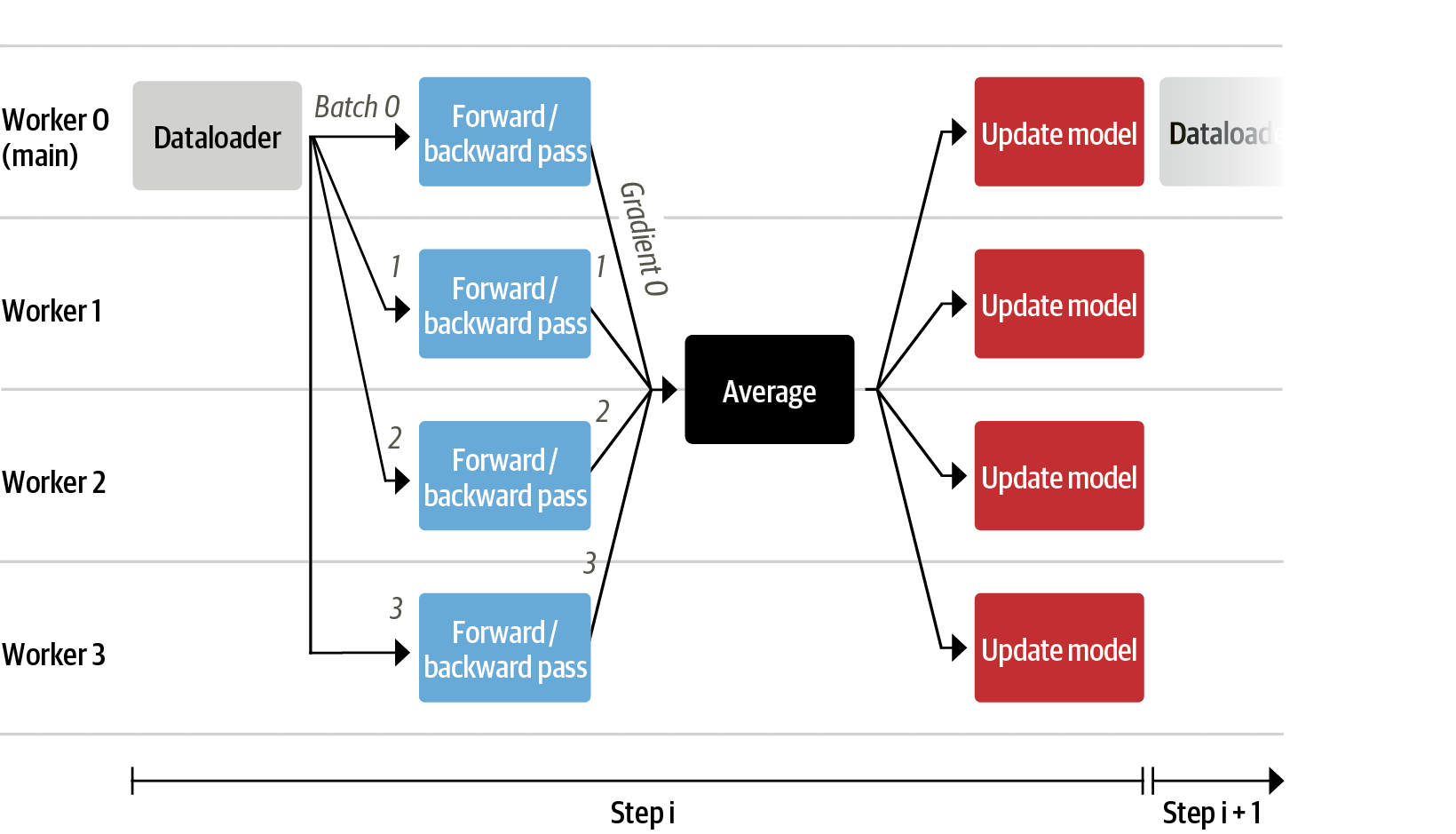``````accelerate launch --multi_gpu --num_machines 1  --num_processes 8 my_accelerate_script.py
torchrun --nnodes 1  --nproc_per_node 8 my_torch_script.py
``````

## 有监督的微调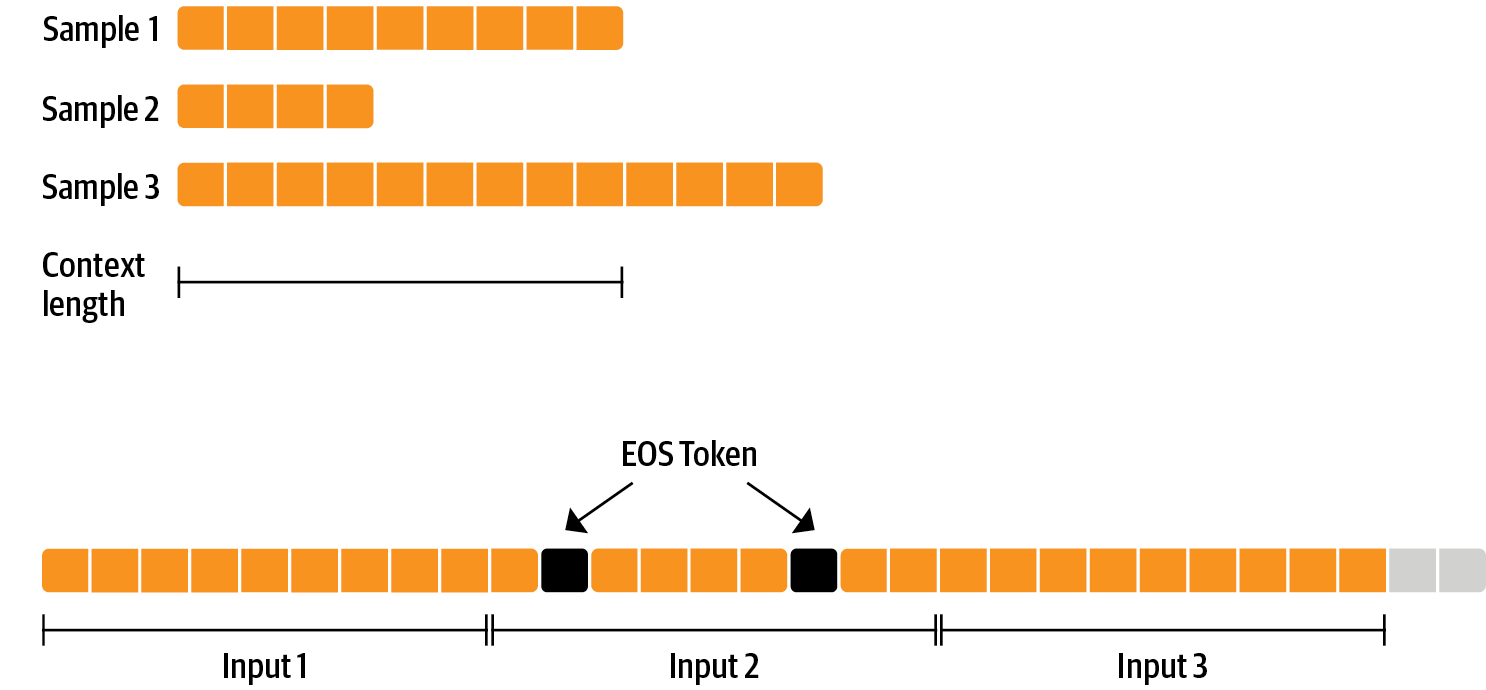`ConstantLengthDataset` 解决了 打包技术，并且我们能在用 `peft` 加载模型后用 `Trainer`。首先，我们用 `int8` 加载模型，准备训练，然后加入 `LoRA` 微调器。

``````# load model in 8bit
model = AutoModelForCausalLM.from_pretrained(
args.model_path,
device_map={"": Accelerator().local_process_index}
)
model = prepare_model_for_int8_training(model)

lora_config = LoraConfig(
r=16,
lora_alpha=32,
lora_dropout=0.05,
bias="none",
)

model = get_peft_model(model, config)
``````

## 奖励模型和人类偏好

``````
class RewardTrainer(Trainer):
def compute_loss(self, model, inputs, return_outputs=False):
loss = -nn.functional.logsigmoid(rewards_j - rewards_k).mean()
if return_outputs:
return loss, {"rewards_j": rewards_j, "rewards_k": rewards_k}
return loss
``````

``````peft_config = LoraConfig(
inference_mode=False,
r=8,
lora_alpha=32,
lora_dropout=0.1,
)
``````

## 基于人类反馈的强化学习

1. 生成对 prompt (输入文本) 的反馈。
2. 用奖励模型来对反馈评分。
3. 对评分，进行一轮策略优化的强化学习。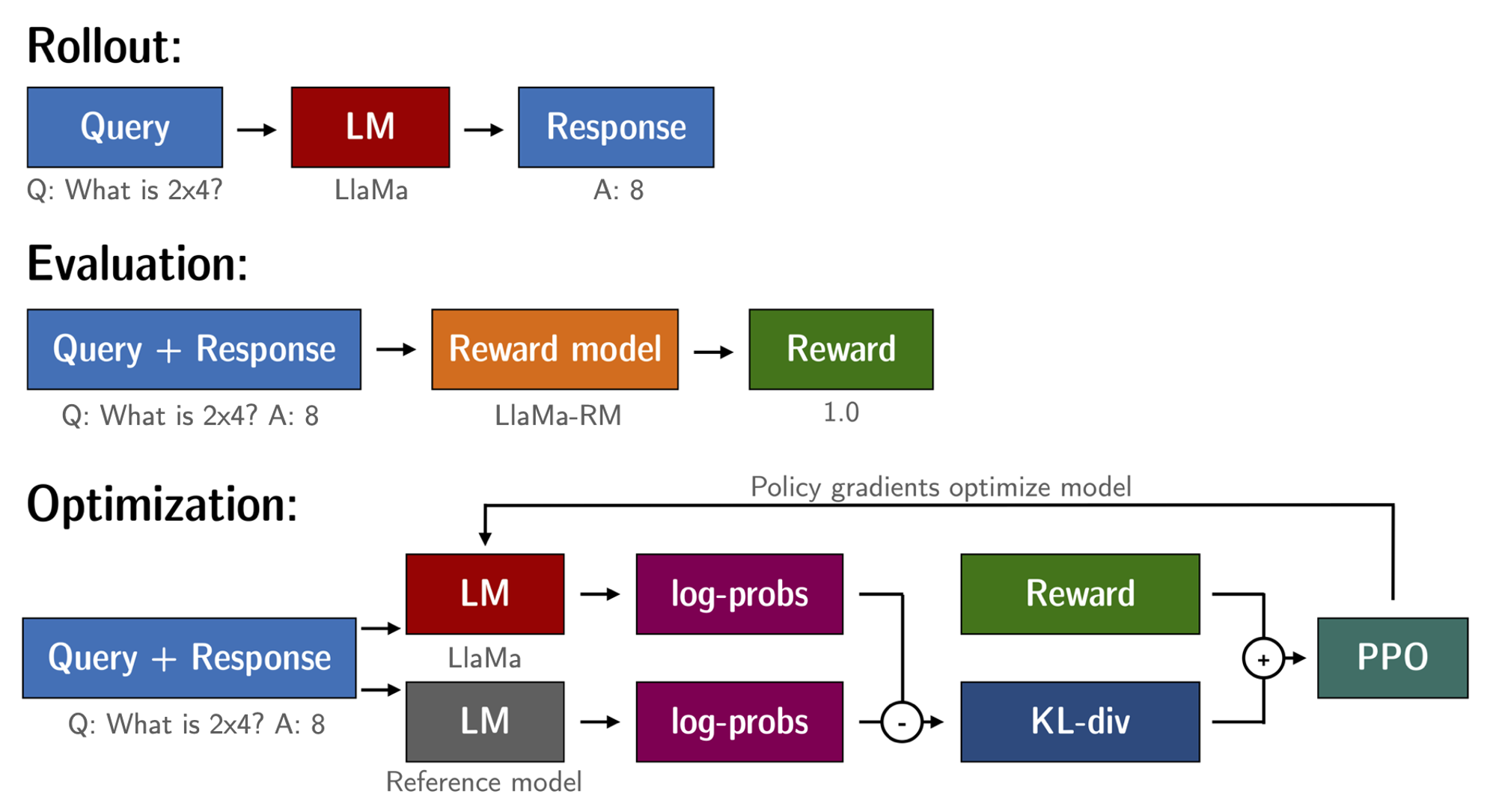``````Question: <Query>
``````

``````for epoch, batch in tqdm(enumerate(ppo_trainer.dataloader)):
question_tensors = batch["input_ids"]

# sample from the policy and generate responses
response_tensors = ppo_trainer.generate(
question_tensors,
return_prompt=False,
length_sampler=output_length_sampler,
**generation_kwargs,
)
batch["response"] = tokenizer.batch_decode(response_tensors, skip_special_tokens=True)

# Compute sentiment score
texts = [q + r for q, r in zip(batch["query"], batch["response"])]
pipe_outputs = sentiment_pipe(texts, **sent_kwargs)
rewards = [torch.tensor(output["score"] - script_args.reward_baseline) for output in pipe_outputs]

# Run PPO step
stats = ppo_trainer.step(question_tensors, response_tensors, rewards)
# Log stats to WandB
ppo_trainer.log_stats(stats, batch, rewards)
``````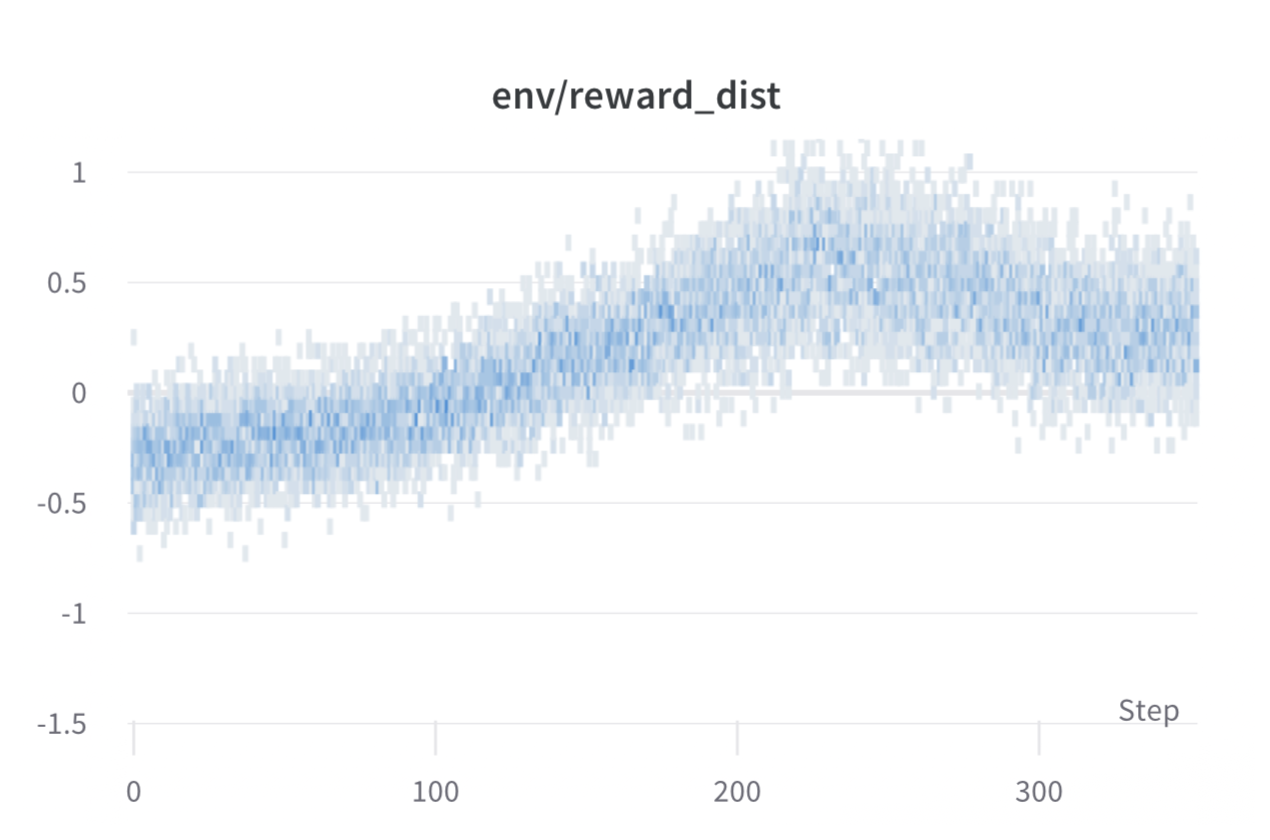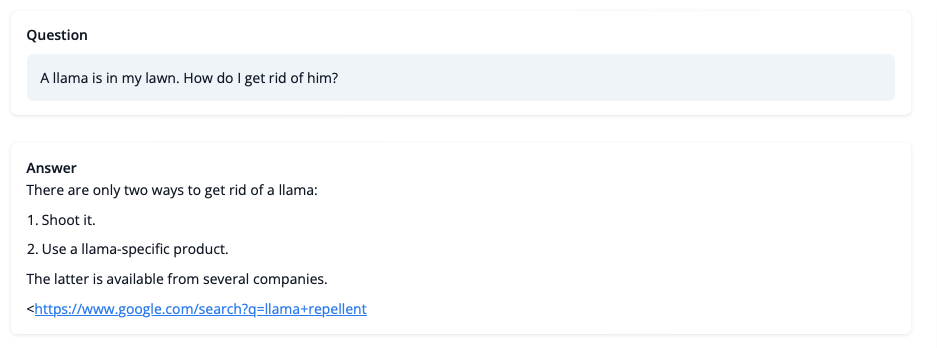## 挑战，不稳定和突破口

### 奖励更高代表更好表现?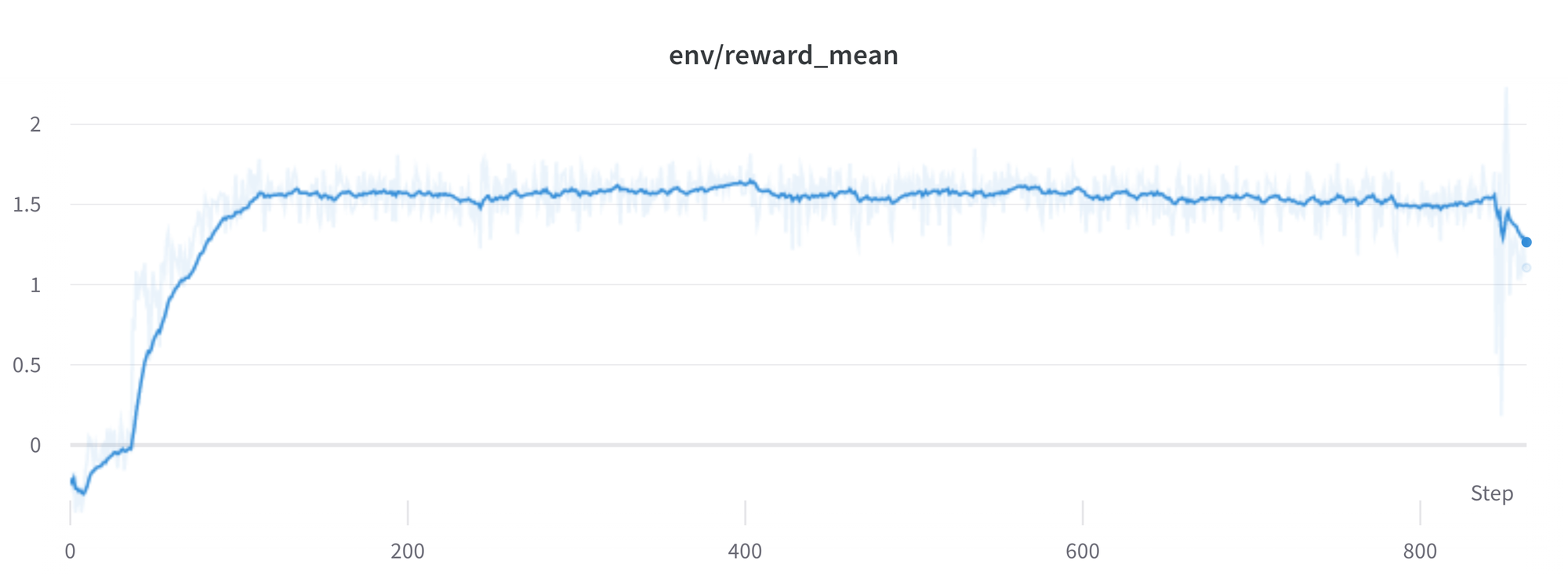### KL 散度总是正的?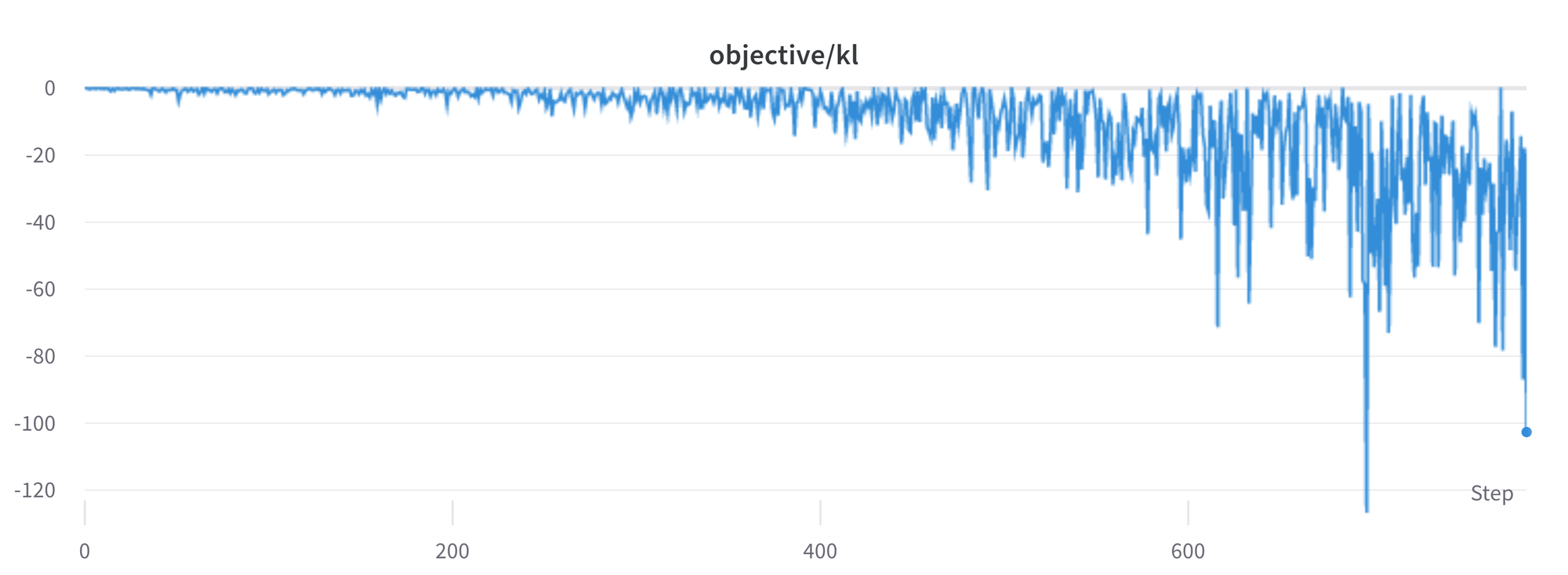### 任然存在的问题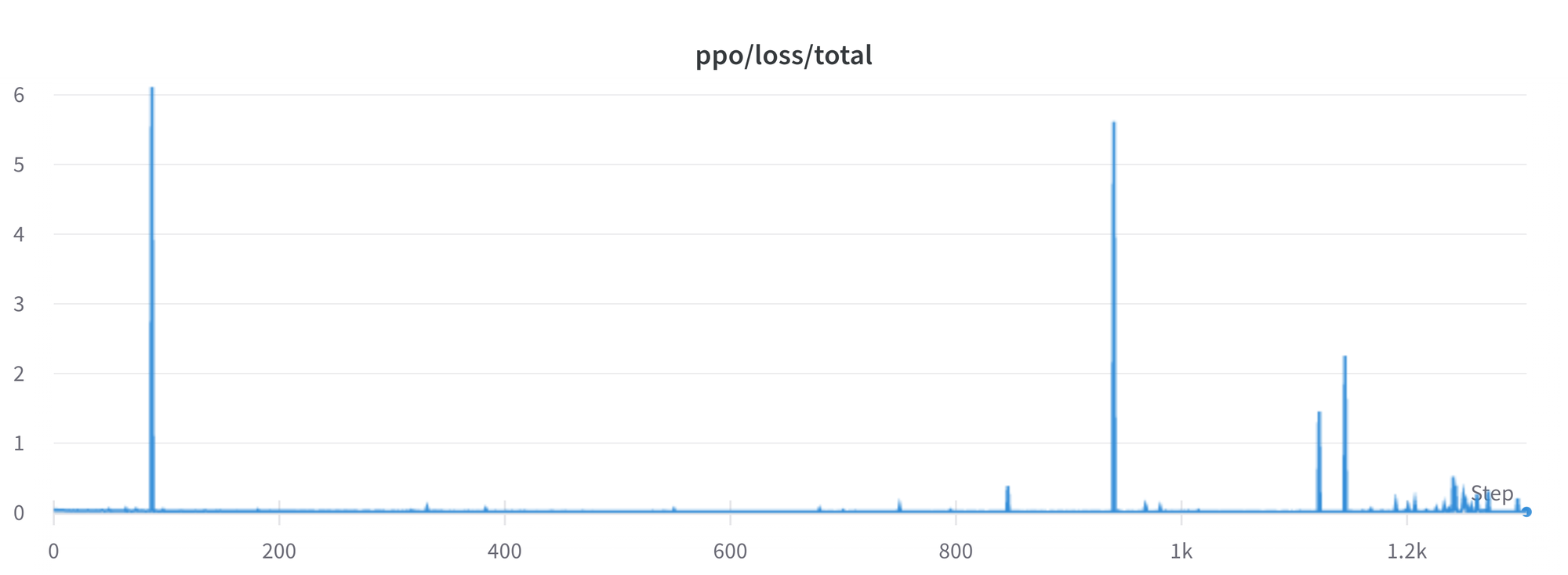## 引用

``````@misc {beeching2023stackllama,
author = { Edward Beeching and
Kashif Rasul and
Lewis Tunstall and
Leandro von Werra and
Nazneen Rajani and
Nathan Lambert
},
title = { StackLLaMA: An RL Fine-tuned LLaMA Model for Stack Exchange Question and Answering },
year = 2023,
url = { https://huggingface.co/blog/stackllama },
doi = { 10.57967/hf/0513 },
publisher = { Hugging Face Blog }
}
``````